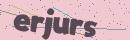# ThinkPHP6全局事务(中间件及订阅事件)

2020-06-19 18:12:00`namespace app\api\base;`

`php think make:middleware BaseResponse`

`namespace app\api\middleware;`

```public function handle(\$request, Closure \$next)
{
\$response = \$next(\$request);
\$this->code = \$response->getCode();
return \$response;
}```

`protected \$code = 0;`

`protected \$middleware = [BaseResponse::class];`

`php think make:event DBSubmit`

```private \$start = false;

{
if (!\$this->start) {
\$this->start = true;
Db::startTrans();
}
}

{
if (\$this->start) {
Db::commit();
}
}

{
if (\$this->start) {
Db::rollback();
}
}

public function subscribe(Event \$event)
{
}```

```use think\Event;

```'subscribe'    =>    [
'app\event\DBSubmit',
// 更多事件订阅
],```

```public function end(\think\Response \$response)
{
if (\$this->code == 200) {
// 提交数据
event('dbCommit');
} else {
// 回滚数据
event('dbRollback');
}
}```

```<?php
namespace app\api\base;

use think\Model;

class BaseModel extends Model
{

}```

```<?php
namespace app\api\base;

use think\Model;

class BaseModel extends Model
{
public static function onBeforeWrite(Model \$model)
{
}

public static function onBeforeUpdate(Model \$model)
{
\$data = array_merge(\$model->getData(), [\$model->updateTime => date('Y-m-d H:i:s')]);
\$model->data(\$data);
}

public static function onBeforeInsert(Model \$model)
{
\$data = array_merge(\$model->getData(), [\$model->createTime => date('Y-m-d H:i:s')]);
\$model->data(\$data);
}

public static function onBeforeRestore(Model \$model)
{
}

public static function onBeforeDelete(Model \$model)
{
}

{
}
}```

```public function render(\$request, Throwable \$e): Response
{
event('dbRollback');
// 添加自定义异常处理机制

// 其他错误交给系统处理
return parent::render(\$request, \$e);
}```

```[database]
hostname = '127.0.0.1';
database = 'test';
hostport = '3306';``````public function test()
{
\$model_test = new Test();
\$model_test->save([
'name' => 1
]);

return \$x;
}```

```return [
'code' => 0,
'msg' => 'msg'
]```

```<?php

namespace app\api\exception;

use think\Response;
use Throwable;

class ApiException extends \think\Exception
{
public static function response(\$request, Throwable \$e): Response
{
\$msg = \$e->getMessage();
\$code = \$e->getCode();
if (empty(\$code)) {
\$code = 999;
}

return json([
'msg' => \$msg,
'code' => \$code
]);
}
}```

```use app\api\exception\ApiException;
use think\Response;

if (!function_exists('errorService')) {

function errorService(\$msg): Response
{
throw new ApiException(\$msg);
}
}```

app 下的 ExceptionHandle 类的 render 方法也要增加一个判断:

```public function render(\$request, Throwable \$e): Response
{
event('dbRollback');
// 添加自定义异常处理机制

if (\$e instanceof ApiException) {
return ApiException::response(\$request, \$e);
}

// 其他错误交给系统处理
return parent::render(\$request, \$e);
}```

```public function test()
{
\$model_test = new Test();
\$model_test->save(
[
'name' => 1
]
);
errorService('msg', 222);
}```